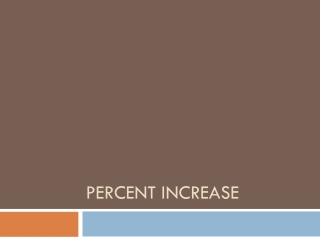DownloadDownload PresentationPercent increase

# Percent increase

Télécharger la présentation## Percent increase

- - - - - - - - - - - - - - - - - - - - - - - - - - - E N D - - - - - - - - - - - - - - - - - - - - - - - - - - -
##### Presentation Transcript

1. Percent increase

2. Percent Change • The ratio comparing a change in a quantity to the original amount. • Percent change= amount of change/original amount

3. Percent Increase • Write an equation • Percent increase = amount of increase/original amount • Write/solve a proportion • Amount of increase/original amount = percent increase (%) / 100

4. Find the percent increase from 12 to 15 • Equation • Amount of increase / original amount = percent increase • 15-12 =3  amount of increase • Original amount = 12 • 3/12 = .25 = 25% • Proportion • Amount of increase / original = percent increase / 100 • 3/12=x/100 • 12x=300 • X=25

5. Find the percent increase from 40 to 45

6. Amount of Profit • Cost- original amount spent for an item • Profit- the money gained when an item is sold at cost • To find the amount of profit: • Multiply the original cost by the percent profit

7. A company buys microwave ovens for \$200 each then sells each at a gain of 15%. What is the company’s profit? • Write an equation • P = .15 x 200 • P = \$30.00 • Write/solve a proportion • Profit / original = percent profit (%) / 100 • P/200 = 15/100 • 3000 = 100p • P = \$30

8. Selling Price • Selling price- the amount for which the item is sold. Add the profit to the cost to find the selling price. • A video dealer imports large flat screen TVs for \$1200 each. The dealer then sells each TV at a profit of 30%. What is the selling price? • SP = \$1200 + (30% of 1200) • SP = \$1200 + (.30 x 1200 = \$360) • SP = \$1200 + \$360 • SP = \$1560

9. Sales tax- amount of tax added to the marked price by state or local government. • Write an equation • Sales tax rate x marked price = sales tax • Set up/solve a proportion • Sales tax / marked price = sales tax rate (%) / 100

10. A calculator is priced at \$29.95 with a sales tax of 6%. What is the amount of sales tax to the nearest cent? • Equation • .06 x 29.95 = 1.797 = \$1.80 • Proportion • t/29.95 = 6/100 • 100t=179.70 • T-1.7970 = \$1.80

11. Discount • Discount = discount rate x list price • Sale price = list price – discount • Discount rate = amount of discount / list price

12. Markup • Markup = list price – wholesale price • Markup rate = markup / wholesale price • Markup rate is expressed as a percent

13. Commission-Amount of money earned for selling goods or services. • Equation • Commission = commission rate x total sales • Write/solve proportion • Commission/total sales =commission rate (%) / 100

14. 6% commission on \$25,000 in sales • .06 x 25,000 = • x/25,000 = 6/100 =

15. 6% commission on \$25,000 in sales • .06 x 25,000 = \$1500 • x/25,000 = 6/100 = \$1500

16. \$1755 earned on \$92,000 in sales • C x 92,000 = 1755 • 1755/92000 = x/100

17. \$1755 earned on \$92,000 in sales • C x 92,000 = 1,755 • C=about 1.9% • 1755/92000 = x/100 • C=about 1.9%

18. Simple Interest • Principal (p)- amount of money borrowed or deposited • Interest (I)- amount earned or paid in exchange for the use of money • Rate of interest (r)- percent of interest earned or paid • Time (t)- how long in years the principal is borrowed or left on deposit. • Simple interest- interest earned or paid only on the principal for a stated period of time

19. Interest = principal x rate x time • I=prt Time is expressed in years or fractional part of a year

20. Find the simple interest (I):\$800 at an annual rate of 8% for 9 months • I=prt • I=(800)(.08)(9/12) • I= \$48

21. Compound Interest • Compound interest- interest paid on the principal and on the interest accumulated to date. • balance